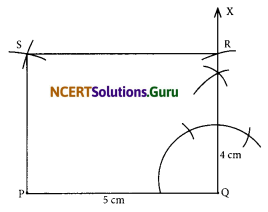# NCERT Solutions for Class 8 Maths Chapter 4 Practical Geometry Ex 4.5

These NCERT Solutions for Class 8 Maths Chapter 4 Practical Geometry Ex 4.5 Questions and Answers are prepared by our highly skilled subject experts.

## NCERT Solutions for Class 8 Maths Chapter 4 Practical Geometry Exercise 4.5

Draw the following.

Question 1.
The square READ with RE = 5.1 cm.
Solution:Steps of construction:
I. Draw a line segment RE = 5.1 cm.
II. At E, draw $$\overrightarrow{\mathrm{EX}}$$, such that ∠REX = 90°.
III. From $$\overrightarrow{\mathrm{EX}}$$, cut-off $$\overrightarrow{\mathrm{EA}}$$ = 5.1 cm.
IV. With centre at A, draw an arc above RE of radius = 5.1 cm.
V. With centre at R, and radius = 5.1 cm, draw another arc to intersect the previous arc at D.
VI. Join DA and DR. Thus, READ is the required square.Question 2.
A rhombus whose diagonals are 5.2 cm and 6.4 cm long.
Solution:Note: The diagonals of a rhombus bisect each other at right angles.
Steps of Construction:
I. Draw a line segment AC = 5.2 cm.
II. Draw $$\overrightarrow{\mathrm{XY}}$$, the perpendicular bisector of AC.
III. From XY, cut-off OD = $$\frac{1}{2}$$ × (6.4 cm) = 3.2 cm.
IV. Similarly, cut-off OB = $$\frac{1}{2}$$ × (6.4 cm) = 3.2 cm.
V. Join AD, DC, CB, and BA. Thus, ABCD is the required rhombus.

Question 3.
A rectangle with adjacent sides of length 5 cm and 4 cm.
Solution:Steps of Construction:
I. Draw a line segment PQ = 5 cm
II. At Q draw $$\overrightarrow{\mathrm{QX}}$$ such that ∠PQX = 90°
III. From $$\overrightarrow{\mathrm{QX}}$$, cut-off QR = 4 cm.
IV. With centre R and radius = 5 cm draw an arc.
V. With centre P and radius = 4 cm draw an arc to intersect the previous arc at S.
VI. Join RQ and RS. Thus, PQRS is the required rectangle.Question 4.
A parallelogram OKAY where OK = 5.5 cm and KA = 4.2 cm. Is it unique?
Solution:Steps of Construction:
I. Draw a line segment OK = 5.5 cm.
II. At K, draw a ray $$\overrightarrow{\mathrm{KX}}$$.
III. From $$\overrightarrow{\mathrm{KX}}$$, cut-off KA = 4.2 cm.
IV. With centre at A and radius = 5.5 cm draw an arc above OK.
V. With centre O and radius = 4.2 cm, draw another arc to intersect the previous arc at Y.
VI. Join OY and AY. Thus, OKAY is the required parallelogram.

error: Content is protected !!